# 回归损失函数1：L1 loss, L2 loss以及Smooth L1 Loss的对比

### 均方误差MSE ($L_2$ Loss)

$MSE = \frac{\sum_{i=1}^n(f_{x_i} - y_i)^2}{n}$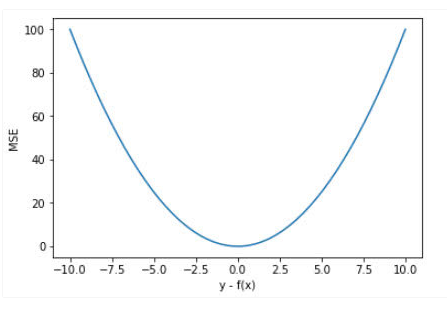MSE的函数曲线光滑、连续，处处可导，便于使用梯度下降算法，是一种常用的损失函数。 而且，随着误差的减小，梯度也在减小，这有利于收敛，即使使用固定的学习速率，也能较快的收敛到最小值。

$y$$f(x)$也就是真实值和预测值的差值大于1时，会放大误差；而当差值小于1时，则会缩小误差，这是平方运算决定的。MSE对于较大的误差（$>1$）给予较大的惩罚，较小的误差（$<1$）给予较小的惩罚。也就是说，对离群点比较敏感，受其影响较大。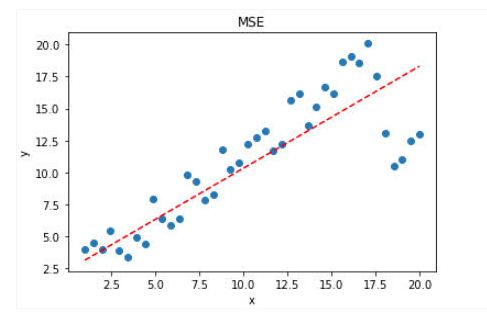### 平均绝对误差($L_1$ Loss)

$MAE = \frac{\sum_{n=1}^n\mid f(x_i) - y_i\mid}{n}$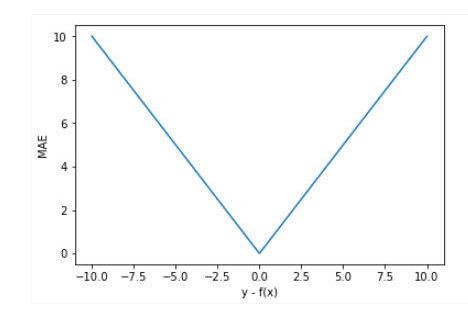MAE曲线连续，但是在$y-f(x)=0$处不可导。而且 MAE 大部分情况下梯度都是相等的，这意味着即使对于小的损失值，其梯度也是大的。这不利于函数的收敛和模型的学习。但是，无论对于什么样的输入值，都有着稳定的梯度，不会导致梯度爆炸问题，具有较为稳健性的解。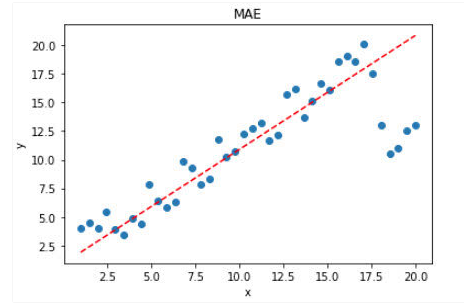### MSE和MAE的选择

• 从梯度的求解以及收敛上，MSE是由于MAE的。MSE处处可导，而且梯度值也是动态变化的，能够快速的收敛；而MAE在0点处不可导，且其梯度保持不变。对于很小的损失值其梯度也很大，在深度学习中，就需要使用变化的学习率，在损失值很小时降低学习率。

• 对离群（异常）值得处理上，MAE要明显好于MSE。

### Smooth $L_1$ Loss

$\text{Smooth}{L_1}(x) =\left \{ \begin{array}{c} 0.5x^2 & if \mid x \mid <1 \\ \mid x \mid - 0.5 & otherwise \end{array} \right.$

Smooth $L_1$ 能从两个方面限制梯度：

1. 当预测框与 ground truth 差别过大时，梯度值不至于过大；
2. 当预测框与 ground truth 差别很小时，梯度值足够小。

#### 对比$L_1$ Loss 和 $L_2$ Loss

\begin{align} L_2(x) &= x^2 \\ L_1(x) &= x \\ smooth_{L_1}(x) &=\left \{ \begin{array}{c} 0.5x^2 & if \mid x \mid <1 \\ \mid x \mid - 0.5 & otherwise \end{array} \right. \end{align}

\begin{align} \frac{\partial L_2(x)}{\partial x} &= 2x \\ \frac{\partial L_1(x)}{\partial x} &= \left \{ \begin{array}{c} 1 & \text{if } x \geq 0 \\ -1 & \text{otherwise} \end{array} \right. \\ \frac{\partial smooth_{L_1}(x)}{\partial x} &=\left \{ \begin{array}{c} x & if \mid x \mid <1 \\ \pm1 & otherwise \end{array} \right. \end{align}

• 根据公式-4，当$x$增大时，$L_2$的损失也增大。 这就导致在训练初期，预测值与 groud truth 差异过于大时，损失函数对预测值的梯度十分大，训练不稳定。

• 根据公式-5,$L_1$$x$的导数为常数，在训练的后期，预测值与ground truth差异很小时，$L_1$的导数的绝对值仍然为1，而 learning rate 如果不变，损失函数将在稳定值附近波动，难以继续收敛以达到更高精度。

• 根据公式-6，$\text{Smotth } L_1$$x$较小时，对$x$的梯度也会变小。 而当$x$较大时，对$x$的梯度的上限为1，也不会太大以至于破坏网络参数。$Smooth L_1$完美的避开了$L_1$$L_2$作为损失函数的缺陷。

$L_1$ Loss ,$L_2$ Loss以及$Smooth L_1$ 放在一起的函数曲线对比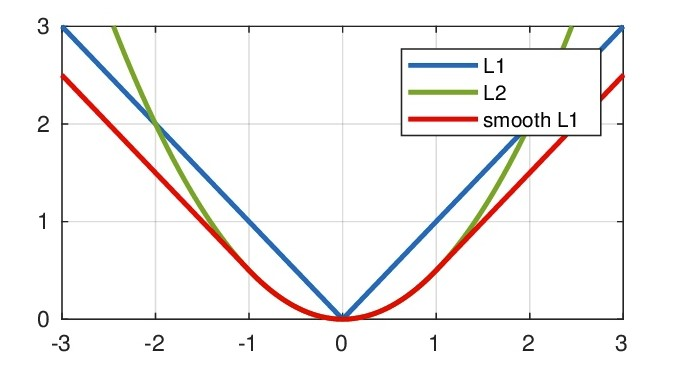#### 实现 (PyTorch)

def _smooth_l1_loss(input, target, reduction='none'):
# type: (Tensor, Tensor) -> Tensor
t = torch.abs(input - target)
ret = torch.where(t < 1, 0.5 * t ** 2, t - 0.5)
if reduction != 'none':
ret = torch.mean(ret) if reduction == 'mean' else torch.sum(ret)
return ret      

def smooth_l1_loss(input, target, beta=1. / 9, reduction = 'none'):
"""
very similar to the smooth_l1_loss from pytorch, but with
the extra beta parameter
"""
n = torch.abs(input - target)
cond = n < beta
ret = torch.where(cond, 0.5 * n ** 2 / beta, n - 0.5 * beta)
if reduction != 'none':
ret = torch.mean(ret) if reduction == 'mean' else torch.sum(ret)
return ret

### 总结

Smooth L1 和 L1 Loss 函数的区别在于，L1 Loss 在0点处导数不唯一，可能影响收敛。Smooth L1的解决办法是在 0 点附近使用平方函数使得它更加平滑。

Smooth L1的优点

• 相比于L1损失函数，可以收敛得更快。
• 相比于L2损失函数，对离群点、异常值不敏感，梯度变化相对更小，训练时不容易跑飞。
posted @ 2019-12-11 11:46  Brook_icv  阅读(...)  评论(...编辑  收藏